• ## TensorFlow神经网络实现股票预测

千次阅读 多人点赞 2020-10-07 23:02:55
神经网络（NeuralNetworks）是一种用训练数据拟合目标函数的黑箱模型，只要数据量足够大，它可以拟合出输入到输出...本篇博文将使用TensorFlow神经网络进行股市的预测，利用数据样本学习，得到相关因素预测股票走势。
目录

1、数据来源

2、数据可视化

3、神经网络设计

神经网络（NeuralNetworks）是一种用训练数据拟合目标函数的黑箱模型，只要数据量足够大，它可以拟合出输入到输出之间的任意函数关系。

本篇博文将使用TensorFlow神经网络进行股市的预测，利用数据样本学习，得到相关因素预测股票走势。

1、数据来源

首先预设一组数据，下图为股票上证指数2019年07月到2019年09月的30天开盘价格和收盘价格。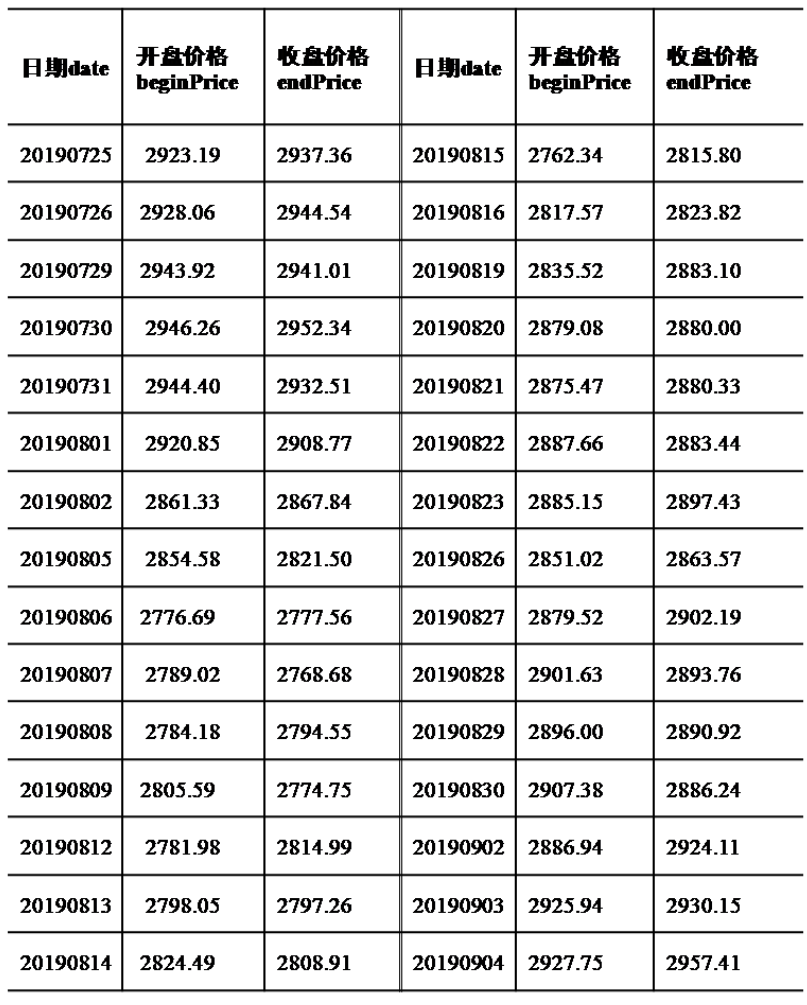数据在程序中实现，代码如下所示：

date = np.linspace(1,30,30)
beginPrice = np.array([2923.19,2928.06,2943.92,2946.26,2944.40,2920.85,2861.33,2854.58,2776.69,2789.02,
2784.18,2805.59,2781.98,2798.05,2824.49,2762.34,2817.57,2835.52,2879.08,2875.47,
2887.66,2885.15,2851.02,2879.52,2901.63,2896.00,2907.38,2886.94,2925.94,2927.75])
endPrice = np.array([2937.36,2944.54,2941.01,2952.34,2932.51,2908.77,2867.84,2821.50,2777.56,2768.68,
2794.55,2774.75,2814.99,2797.26,2808.91,2815.80,2823.82,2883.10,2880.00,2880.33,
2883.44,2897.43,2863.57,2902.19,2893.76,2890.92,2886.24,2924.11,2930.15,2957.41])

2、数据可视化

基于matplotlib可视化库，建立一个30行2列的矩阵存储股票数据，矩阵的第一列输入上列数据中的股票开盘价格，第二列输入股票的收盘价格，如果股票的收盘价格高于开盘价格则用红色显示，反之则用绿色显示，可视化股票数据如下图所示。采用本实例所设计的神经网络预测股票收盘均价，并可视化预测结果。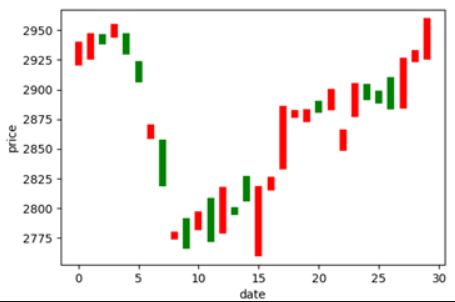使用前需要安装matplotlib模块，指令如下所示：

pip install matplotlib

实现代码如下所示：

for i in range(0,30):  # 画柱状图
dateOne = np.zeros()
dateOne = i;
dateOne = i;
priceOne = np.zeros()
priceOne = beginPrice[i]
priceOne = endPrice[i]
if endPrice[i]>beginPrice[i]:
plt.plot(dateOne,priceOne,'r',lw=6)
else:
plt.plot(dateOne,priceOne,'g',lw=6)
plt.xlabel("date")
plt.ylabel("price")

3、神经网络设计

基于TensorFlow框架，设计三层神经网络，隐含层包括25个节点，利用所设计的神经网络来预测股票的收盘均价。使用前需要安装TensorFlow模块，指令如下所示：

pip install tensorflow

【拓展】4行指令解决pip下载Python第三方库太慢问题（pip更换国内下载源）

实现代码如下所示：

dateNormal = np.zeros([30,1])
priceNormal = np.zeros([30,1])
#归一化
for i in range(0,30):
dateNormal[i,0] = i/29.0;
priceNormal[i,0] = endPrice[i]/3000.0;
x = tf.placeholder(tf.float32,[None,1])
y = tf.placeholder(tf.float32,[None,1])
# X->hidden_layer
w1 = tf.Variable(tf.random_uniform([1,25],0,1))
b1 = tf.Variable(tf.zeros([1,25]))
wb1 = tf.matmul(x,w1)+b1
layer1 = tf.nn.relu(wb1) # 激励函数
# hidden_layer->output
w2 = tf.Variable(tf.random_uniform([25,1],0,1))
b2 = tf.Variable(tf.zeros([30,1]))
wb2 = tf.matmul(layer1,w2)+b2
layer2 = tf.nn.relu(wb2)
loss = tf.reduce_mean(tf.square(y-layer2)) #y为真实数据， layer2为网络预测结果
#梯度下降
with tf.Session() as sess:
sess.run(tf.global_variables_initializer())
for i in range(0,20000):
sess.run(train_step,feed_dict={x:dateNormal,y:priceNormal})
#预测， X  w1w2 b1b2 -->layer2
pred = sess.run(layer2,feed_dict={x:dateNormal})
predPrice = np.zeros([30,1])
for i in range(0,30):
predPrice[i,0]=(pred*3000)[i,0]
plt.plot(date,predPrice,'b',lw=1)
plt.show()

运行以上代码可视化神经网络的预测结果如下图所示：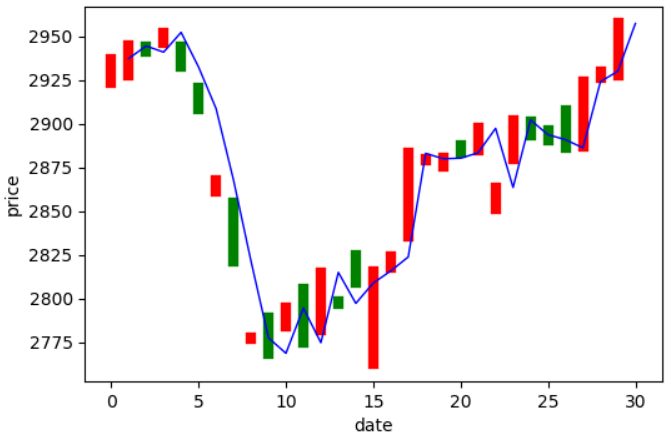关注公众号，回复关键字：股票预测，获取项目源码~

展开全文• 神经网络（NN）它是一种模仿动物神经网络行为特征...本文分享使用Tensorflow神经网络进行股市的预测 1、数据来源 首先找到一组股票数据，数据可以网络上爬虫，东方财富、大智慧都有。爬虫方法参看以前的文章。 .
神经网络（NN）它是一种模仿动物神经网络行为特征，进行分布式并行信息处理的算法数学模型。这种网络依靠系统的复杂程度，通过调整内部大量节点之间相互连接的关系，从而达到处理信息的目的。在提供数据量足够大情况下，神经网络可以拟合出输入到输出之间的任意函数关系。

Tensorflow是一个优秀的深度学习框架，具体有啥好处，可以百度了解哈。

本文分享使用Tensorflow神经网络进行股市的预测

1、数据来源

首先找到一组股票数据，数据可以网络上爬虫，东方财富、大智慧都有。爬虫方法参看以前的文章。

date = np.linspace(1, 30, 30)  #beginPrice = np.array([2923.19, 2928.06, 2943.92, 2946.26, 2944.40, 2920.85, 2861.33, 2854.58, 2776.69, 2789.02,                       2784.18, 2805.59, 2781.98, 2798.05, 2824.49, 2762.34, 2817.57, 2835.52, 2879.08, 2875.47,                       2887.66, 2885.15, 2851.02, 2879.52, 2901.63, 2896.00, 2907.38, 2886.94, 2925.94, 2927.75])endPrice = np.array([2937.36, 2944.54, 2941.01, 2952.34, 2932.51, 2908.77, 2867.84, 2821.50, 2777.56, 2768.68,                     2794.55, 2774.75, 2814.99, 2797.26, 2808.91, 2815.80, 2823.82, 2883.10, 2880.00, 2880.33,                     2883.44, 2897.43, 2863.57, 2902.19, 2893.76, 2890.92, 2886.24, 2924.11, 2930.15, 2957.41])

2、数据展示

基于matplotlib可视化库，建立一个30行2列的矩阵存储股票数据，矩阵的第一列是股票开盘价格，第二列是股票的收盘价格，如果股票的收盘价格高于开盘价格则用红色显示，反之则用绿色显示，可视化股票数据如下图所示。

for i in range(0, 30):  # 画柱状图    dateOne = np.zeros()    dateOne = i    dateOne = i    priceOne = np.zeros()    priceOne = beginPrice[i]    priceOne = endPrice[i]    if endPrice[i] > beginPrice[i]:        plt.plot(dateOne, priceOne, 'r', lw=6)    else:        plt.plot(dateOne, priceOne, 'g', lw=6)plt.xlabel("date")plt.ylabel("price")plt.show()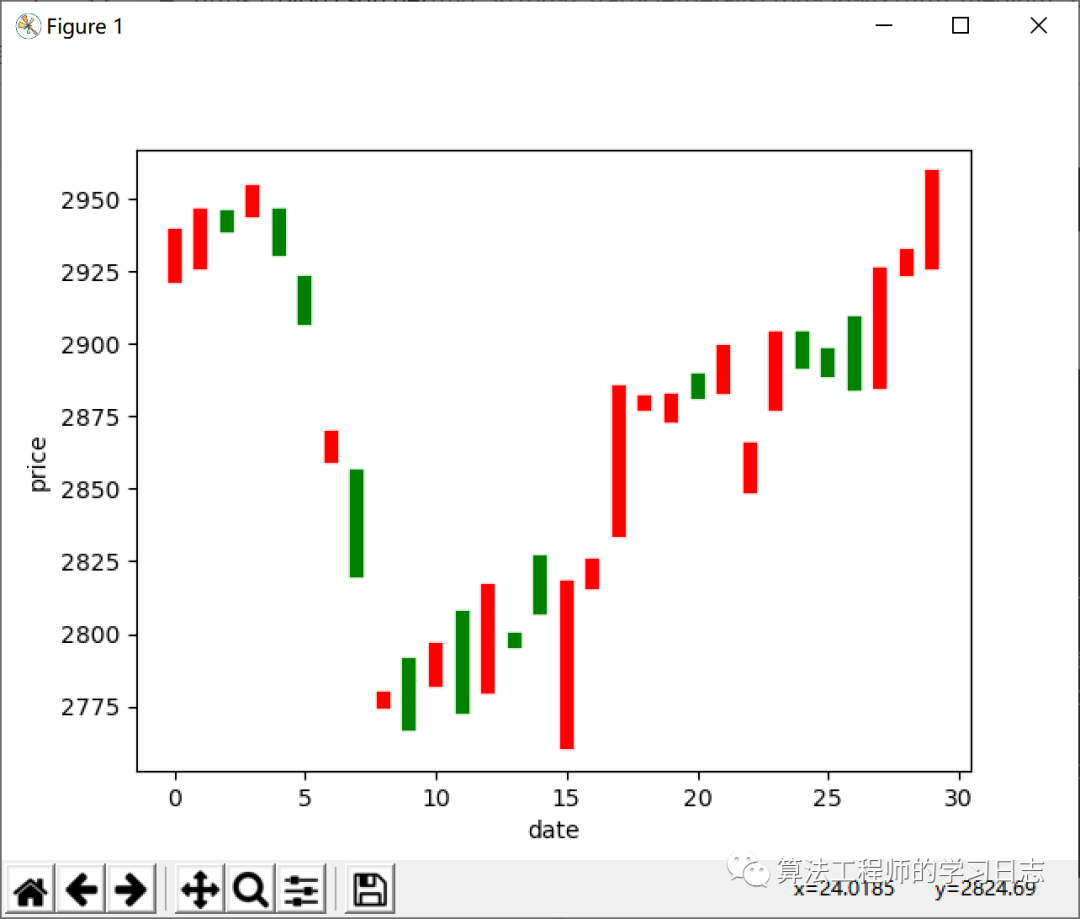3、Tensorflow预测

基于Tensorflow神经网络框架，设计了三层神经网络，其中隐含层包括25个节点，设计的神经网络用来预测股票的收盘价。

dateNormal = np.zeros([30, 1])priceNormal = np.zeros([30, 1])# 归一化for i in range(0, 30):    dateNormal[i, 0] = i / 29.0    priceNormal[i, 0] = endPrice[i] / 3000.0​x = tf.placeholder(tf.float32, [None, 1])y = tf.placeholder(tf.float32, [None, 1])# X->hidden_layerw1 = tf.Variable(tf.random_uniform([1, 25], 0, 1))b1 = tf.Variable(tf.zeros([1, 25]))wb1 = tf.matmul(x, w1) + b1layer1 = tf.nn.relu(wb1)  # 激励函数# hidden_layer->outputw2 = tf.Variable(tf.random_uniform([25, 1], 0, 1))b2 = tf.Variable(tf.zeros([30, 1]))wb2 = tf.matmul(layer1, w2) + b2layer2 = tf.nn.relu(wb2)loss = tf.reduce_mean(tf.square(y - layer2))  # y为真实数据， layer2为网络预测结果# 梯度下降train_step = tf.train.GradientDescentOptimizer(0.1).minimize(loss)with tf.Session() as sess:    sess.run(tf.global_variables_initializer())    for i in range(0, 20000):        sess.run(train_step, feed_dict={x: dateNormal, y: priceNormal})    # 预测， X  w1w2 b1b2 -->layer2    pred = sess.run(layer2, feed_dict={x: dateNormal})    date1 = np.linspace(0, 29, 30)  #    plt.plot(date1, pred*3000, 'b', lw=3)​plt.show()

运行以上代码可视化神经网络的预测结果如下图所示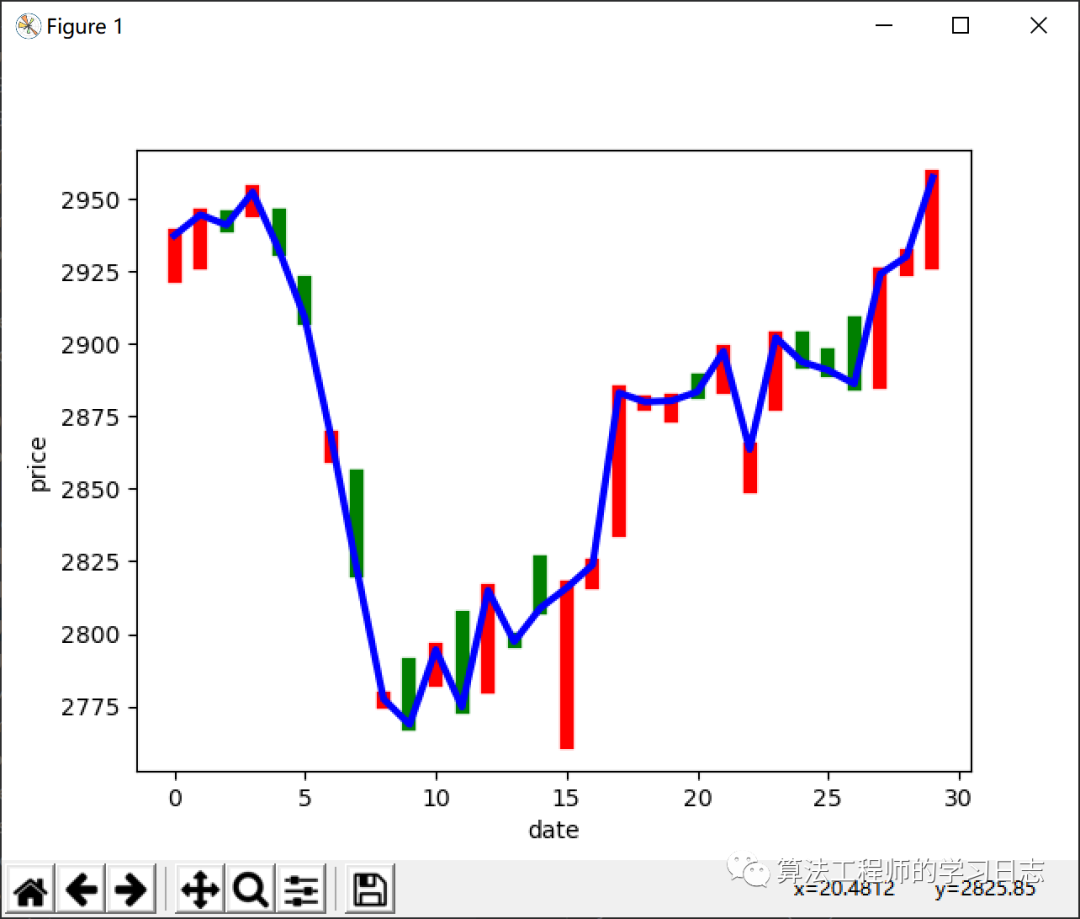完整的代码如下：

import numpy as npimport matplotlib.pyplot as pltimport tensorflow as tf# import tensorflow.compat.v1 as tf# tf.disable_v2_behavior() # 如果是tensorflow2版本就取消这行注释​date = np.linspace(1, 30, 30)  #beginPrice = np.array([2923.19, 2928.06, 2943.92, 2946.26, 2944.40, 2920.85, 2861.33, 2854.58, 2776.69, 2789.02,                       2784.18, 2805.59, 2781.98, 2798.05, 2824.49, 2762.34, 2817.57, 2835.52, 2879.08, 2875.47,                       2887.66, 2885.15, 2851.02, 2879.52, 2901.63, 2896.00, 2907.38, 2886.94, 2925.94, 2927.75])endPrice = np.array([2937.36, 2944.54, 2941.01, 2952.34, 2932.51, 2908.77, 2867.84, 2821.50, 2777.56, 2768.68,                     2794.55, 2774.75, 2814.99, 2797.26, 2808.91, 2815.80, 2823.82, 2883.10, 2880.00, 2880.33,                     2883.44, 2897.43, 2863.57, 2902.19, 2893.76, 2890.92, 2886.24, 2924.11, 2930.15, 2957.41])​for i in range(0, 30):  # 画柱状图    dateOne = np.zeros()    dateOne = i    dateOne = i    priceOne = np.zeros()    priceOne = beginPrice[i]    priceOne = endPrice[i]    if endPrice[i] > beginPrice[i]:        plt.plot(dateOne, priceOne, 'r', lw=6)    else:        plt.plot(dateOne, priceOne, 'g', lw=6)plt.xlabel("date")plt.ylabel("price")# plt.show()​​dateNormal = np.zeros([30, 1])priceNormal = np.zeros([30, 1])# 归一化for i in range(0, 30):    dateNormal[i, 0] = i / 29.0    priceNormal[i, 0] = endPrice[i] / 3000.0​x = tf.placeholder(tf.float32, [None, 1])y = tf.placeholder(tf.float32, [None, 1])# X->hidden_layerw1 = tf.Variable(tf.random_uniform([1, 25], 0, 1))b1 = tf.Variable(tf.zeros([1, 25]))wb1 = tf.matmul(x, w1) + b1layer1 = tf.nn.relu(wb1)  # 激励函数# hidden_layer->outputw2 = tf.Variable(tf.random_uniform([25, 1], 0, 1))b2 = tf.Variable(tf.zeros([30, 1]))wb2 = tf.matmul(layer1, w2) + b2layer2 = tf.nn.relu(wb2)loss = tf.reduce_mean(tf.square(y - layer2))  # y为真实数据， layer2为网络预测结果# 梯度下降train_step = tf.train.GradientDescentOptimizer(0.1).minimize(loss)with tf.Session() as sess:    sess.run(tf.global_variables_initializer())    for i in range(0, 20000):        sess.run(train_step, feed_dict={x: dateNormal, y: priceNormal})    # 预测， X  w1w2 b1b2 -->layer2    pred = sess.run(layer2, feed_dict={x: dateNormal})    date1 = np.linspace(0, 29, 30)  #    plt.plot(date1, pred*3000, 'b', lw=3)​plt.show()

代码中需要用到numpy、matplotlib和tensorflow三个库，为了提高下载速度，建议切换到国内的pip源，例如豆瓣、清华等

pip install numpy -i https://pypi.tuna.tsinghua.edu.cn/simplepip install matplotlib -i https://pypi.tuna.tsinghua.edu.cn/simplepip install tensorflow -i https://pypi.tuna.tsinghua.edu.cn/simple展开全文python
• Tensorflow神经网络可视化工具Tensorboard简介使用方法 简介 Tensorboard是Tensorflow自带的一款可视化工具，功能强大，可以查看训练过程的loss、学习率变化等，也可以查看网络的结构，这里我们用于查看网络的输入...
Tensorflow神经网络可视化工具Tensorboard简介使用方法
简介
Tensorboard是Tensorflow自带的一款可视化工具，功能强大，可以查看训练过程的loss、学习率变化等，也可以查看网络的结构，这里我们用于查看网络的输入输出的节点
使用方法
import tensorflow as tf
from tensorflow.python.platform import gfile

# 这是从二进制格式的pb文件加载模型
graph = tf.get_default_graph()
graphdef = graph.as_graph_def()
_ = tf.import_graph_def(graphdef, name="")

#这是从文件格式的meta文件加载模型
_ = tf.train.import_meta_graph("model.ckpt.meta")
summary_write = tf.summary.FileWriter("/data/TensorFlowAndroidMNIST/logdir" , graph)

然后再启动tensorboard：
tensorboard --logdir /data/TensorFlowAndroidMNIST/logdir --host 你的ip --port 你端口(默认6006)

我们会在目标路径下看见生成一个文件：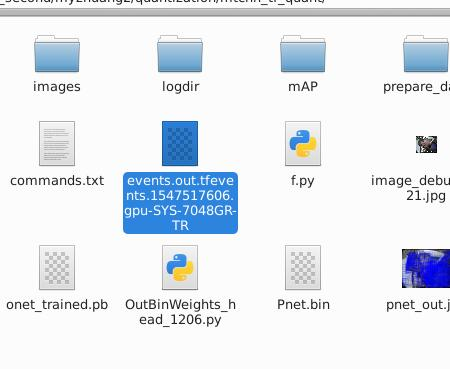然后我们就可以在浏览器打开生成的链接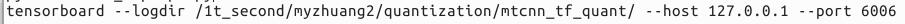展开全文• ## 构建第一个tensorflow神经网络

万次阅读 多人点赞 2019-03-02 22:06:42
大家已经学过如何将待预测数据输入到神经网络中，也明白了神经网络是如何对这些数据进行预测的，还知道了神经网络是如何判断自己预测得是否准确的。那么如果结果预测得不准确，是不是要想办法让预测变得准确呢？这个...
朋友们，如需转载请标明出处：https://blog.csdn.net/jiangjunshow
本篇文章我会教大家构建第一个tensorflow程序。
PS:因为本篇文章是我在人工智能教学中给我的二年级学生写的，我已经教会了他们一定的python和人工智能基础知识， 如果你还不具备这些知识，那么可能会看不懂本篇文章。
下面我们通过一个简单的例子来认识tensorflow的基本用法——使用tensorflow来寻找一个w值，这个值可以使函数J(w)=w2-10w+25的结果最小——就像我们训练神经网络时，寻找参数w和b的值来让成本函数的值最小一样。上面的函数其实等价于J(w)= (w-5)2，这个知识点我们小学或者初中时应该就学过了，有些同学可能忘记了。从上面的式子中很容易可以看出当w等于5时函数J的值是最小的。当然，tensorflow是看不出来的，它需要使用梯度下降算法来一步一步地寻找这个让J最小的w值。
就像在python中使用numpy工具库一样，要想在python中使用tensorflow，第一步就是要将其导入。如下所示，将tensorflow导入并且取名为tf，在后面通过tf就可以调用tensorflow的相关功能了（我们的开发环境依然使用Jupyter Notebook）。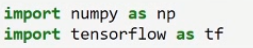首先，我们用下面的语句定义了一个类型为float32的tensorflow变量w。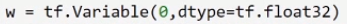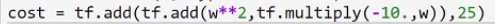下面我们定义了一个梯度下降的实例对象，它的学习率我们将其设为0.01。然后用这个梯度下降对象来最小化cost函数，也就是来寻找让cost函数最小化的w值。我们把上面这些操作用train变量来表示，也就是说，train变量就代表了上面这些操作。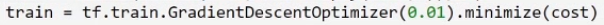下面这三行代码可以说是tensorflow固定的表达形式。大家先不用理解他们。只需要知道通过这三行代码就创建一个tensorflow的session对象了，后面就可以通过这个session对象来让tensorflow执行一些操作。例如下面我们用session.run(w)来获取w变量当前的值，然后用print把这个值打印出来。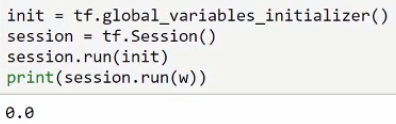因为上面我们只是定义了一些操作，还并没有执行这些操作。所以上面打印出来的w的值还是初始值0。下面我们将使用session对象来执行一系列操作，来寻找使cost函数最小化的w的值。
下面我们用session对象来执行一次train操作，也就是执行一次梯度下降操作；然后再将w值打印出来。在经过了一次梯度下降之后，w的值发生了变化。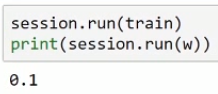下面我们再用session对象来执行1000次梯度下降，并把w的值打印出来。可以看到，经过了1000次梯度下降之后，w的值已经非常接近理想值了（前面我们说过，5可以使cost函数的值最小）。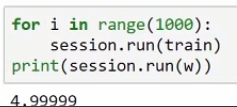通过上面这些代码，你应该对tensorflow的语法有了一些大致的了解。但是对于他们还不太理解，没有关系，通过对教程后面实战编程的学习后，你对他们的理解程度会越来越深入。
在上面的代码中，我们并没有定义梯度下降的反向传播操作，但是梯度下降却成功的执行了，并且还找到了最小值处。这是为什么呢？因为tensorflow在内部它会自动构建反向传播的相关操作。所以以后我们只需要定义神经网络的前向传播操作就可以了，tensorflow框架会根据前向传播自动生成反向传播操作。相当的强大！相当的方便！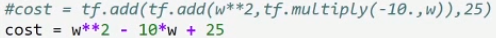下面我再给大家介绍一个tensorflow中非常重要的功能——placehold——占位符功能。我们在训练神经网络时，除了有参数w和b外，还有一个很重要的成员——输入的训练数据。上面cost函数的系数就相当于输入的训练数据的角色。上面cost函数的系数是固定的，分别是1，-10，25。但是在实际应用中，输入数据是变化的。比如我们每一个子训练集都不同。tensorflow的placehold功能可以完美应对这种情况。
我们可以用下面这行代码来定义一个占位符变量x，它是一个维度为(3，1)的float32向量。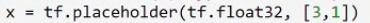然后我们将前面的cost函数的3个系数分别换成上面的占位符变量x中的3个元素。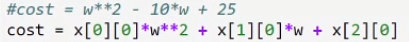上面cost函数中的占位符变量中还并没有实际的数值，正如其名，它只是占了一个位置而已。当整个神经网络构建好了之后，我们可以往这些位置里面填充不同的数值。
比如我们可以先通过下面的语句构建一个与上面占位符维度相同的向量，这个向量里面是有实际数值的，这些数值与我们最原始的cost函数的系数是一样的。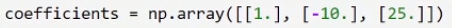然后通过下面的语句将上面的数值向量coefficients填充到我们的占位符x中。这样一来，我们就通过占位符的形式，实现了与前面固定系数时一样的功能了。因为我们往占位符中填充的数值与前面的固定系数是一样的。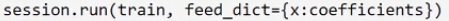重新执行这些代码后，可以看到结果与固定系数时一样。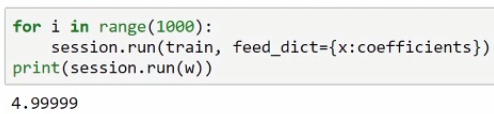当然，有了占位符这个功能，我们就可以往构建好的神经网络中填充不同的数据了，非常的方便。比如我们可以改成填充下面的数值。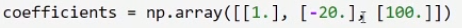重新执行代码后，结果是9.9998，这个结果是非常棒的了。因为对于新的系数来说，w等于10的时候，cost函数的值是最小的。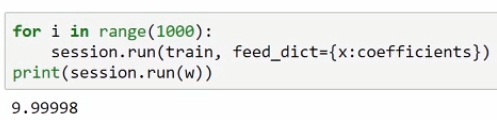下面我把最终完整的代码一次性贴出来。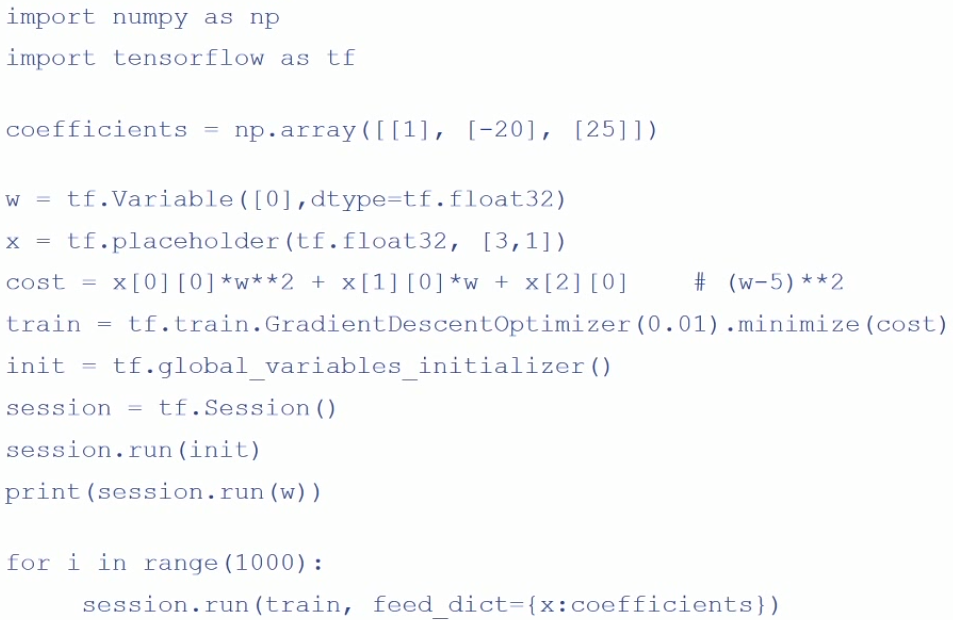有了占位符，我们就可以在执行mini-batch梯度下降时方便地往构建好的神经网络中填充不同的子训练集数据了。
看了本篇文章后，你可能对上面的一些语法还有些陌生。在接下来的实战编程中，我会带领大家进一步熟悉tensorflow，并用它来轻松地构建出非常复杂的神经网络，实战编程中的代码都可以下载运行。


展开全文深度学习 人工智能
• 既然大家看到用TensorFlow实现深层神经网络，想必对BP神经网络的数学理论有了一个大体的认识，所以本文不会对基础知识进行深入讲解，只是对基础知识进行整理和总结，起画龙点睛、增加理解深度的作用。
• 使用TensorFlow对一维电机振动信号检测（输入的信号经过了小波变换，8个特征）机器学习
• 借助 tensorboard 实现tensorflow中定义的深度神经网络可视化。 在程序中实现网络可视化，只需要在加载网络之后，加上这一句： summary_writer = tf.summary.FileWriter('./log/', sess.graph) 上边的 sess....
• 微信公众号：数据挖掘与分析学习 1.导入所需的库 ... from tensorflow.examples.tutorials.mnist import input_data import tensorflow as tf 2.数据准备 mnist=input_data.read_...
• LSTM作为门控循环神经网络因此我们从门控单元切入理解。主要包括： 输入门：It 遗忘门：Ft 输出门：Ot 候选细胞：~Ct 细胞：Ct 隐含状态：Ht 假设隐含状态长度为h，数据Xt是一个样本数为n、特征向量维度为x的批量...LSTM
• 神经网络模型优化的过程中，会遇到许多问题，比如如何设置学习率的问题，我们可通过指数衰减的方式让模型在训练初期快速接近较优解，在训练后期稳定进入最优解区域；针对过拟合问题，通过正则化的方法加以应对；...学习率 过拟合 正则化 滑动平均模型
• 受到生物神经网络的启发 发展历史 生物神经网络单元 逻辑运算单元：and、or、xor等运算 感知机(perceptron)：hw(x)=step(wT⋅x)h_w(x)=step(w^T \cdot x) 多层感知机和反向传播(multi-perceptron and ...BP算法
• # -*- coding: utf-8 -*- # @Time : 2018/3/7 12:43 # @Author : timothy ... 一个完整的tensorflow demo input layer nodes num: 2 hidden layer nodes num: 3 output layer nodes num: 1 数据集：n...
• 用的是TensorFlow2.1 ，在学卷积网络热力图可视化遇到了问题： 网上教程都是用的VGG16， 但想用自己的简单的网络试试，当预测后样本， ` preds = model.predict(x) layer = model.get_layer(‘conv2d’) ...
• import tensorflow as tf from numpy.random import RandomState # 定义训练数据batch大小 ...# 定义神经网络的参数 w1 = tf.Variable(tf.random_normal([2, 3], stddev=1, seed=1)) w2 =数据挖掘
• 引言使用TensorFlow训练好的神经网络模型，来进行对输入数据的输出预测，即软测量的过程。1、训练神经网络模型这里写代码片
• 多层感知机简介 多层感知机模型 这里定义含有两个隐含层的模型，隐含层输出均为256个节点，输入784（MNIST数据集图片大小28*28），输出10。...Tensorflow实现多层感知机 from __future__ import pri...多层感知机
• import pandas as pd def make_data(): col_names = ["ID","K1K2驱动信号","电子锁驱动信号","急停信号","门禁信号","THDV-M"...,nam
• 已知股票的「开盘价」和「收盘价」，利用神经网络来预测「收盘均价」 2.数据源： 日期（data）：[ 1. 2. 3. 4. 5. 6. 7. 8. 9. 10. 11. 12. 13. 14. 15.] 开盘价（beginPrice）：[2438.71,2500.88,2534.95,2512.52...
• 这里搭建了一个入门的神经网络 详细的神经网络搭建过程都在注释里面 主要有3大步 1、定义神经网络的输入，参数和输出，定义前向传播过程 2、定义损失函数及反向传播方法 3、生成会话。训练多少轮 import ...
• Tensorboard专用于展示Tensorflow图的结构及绘制计算结果图像.帮助理解Tensorflow的工作原理,降低学习难度,总之,好工具. 2 基本用法 2.1 直接绘图 Demo import tensorflow as tf LOG_DIR = &quot;./logs/add...可视化
• 人工智能三大学派。TensorFlow（非Keras API）搭建神经网络
• 这个预测很简陋，就是用一个人的高数1、2的两个成绩预测线性代数成绩，数据量不大，预测的实际意义也不大。主要是体验下流程，应付下作业。(￣y▽,￣)╭ 首先是数据的类型，我们这次拿到的数据是3个班的高数1、2和...大数据
• 1 载入Tensorflow模型 import tensorflow as tf import numpy as np import matplotlib.pyplot as plt x_data = np.linspace(-1, 1, 250, dtype=np.float32)[:, np.newaxis] noise = np.random.normal(0, ...迁移学习...